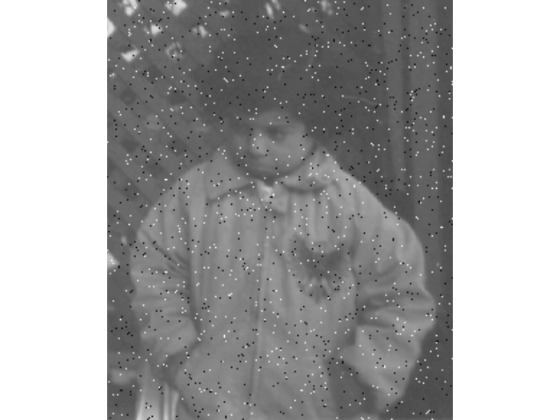# immse

Mean-squared error

## Syntax

``err = immse(X,Y)``

## Description

example

````err = immse(X,Y)` calculates the mean-squared error (MSE) between the arrays `X` and `Y`. A lower MSE value indicates greater similarity between `X` and `Y`.```

## Examples

collapse all

```ref = imread('pout.tif'); imshow(ref)```Create another image by adding noise to a copy of the reference image.

```A = imnoise(ref,'salt & pepper', 0.02); imshow(A)```Calculate mean-squared error between the two images.

```err = immse(A, ref); fprintf('\n The mean-squared error is %0.4f\n', err);```
``` The mean-squared error is 353.7631 ```

## Input Arguments

collapse all

Input array, specified as a numeric array of any dimension.

Data Types: `single` | `double` | `int8` | `int16` | `int32` | `uint8` | `uint16` | `uint32`

Input array, specified as a numeric array of the same size and data type as `X`.

Data Types: `single` | `double` | `int8` | `int16` | `int32` | `uint8` | `uint16` | `uint32`

## Output Arguments

collapse all

Mean-squared error, returned as a positive number. The data type of `err` is `double` unless the input arguments are of data type `single`, in which case `err` is of data type `single`

Data Types: `single` | `double`

## Version History

Introduced in R2014b